xEncyclopedia
In logic
Logic
In philosophy, Logic is the formal systematic study of the principles of valid inference and correct reasoning. Logic is used in most intellectual activities, but is studied primarily in the disciplines of philosophy, mathematics, semantics, and computer science...

, proof by contradiction is a form of proof
Mathematical proof
In mathematics, a proof is a convincing demonstration that some mathematical statement is necessarily true. Proofs are obtained from deductive reasoning, rather than from inductive or empirical arguments. That is, a proof must demonstrate that a statement is true in all cases, without a single...

that establishes the truth or validity
Validity
In logic, argument is valid if and only if its conclusion is entailed by its premises, a formula is valid if and only if it is true under every interpretation, and an argument form is valid if and only if every argument of that logical form is valid....

of a proposition
Proposition
In logic and philosophy, the term proposition refers to either the "content" or "meaning" of a meaningful declarative sentence or the pattern of symbols, marks, or sounds that make up a meaningful declarative sentence...

by showing that the proposition's being false would imply a contradiction
In classical logic, a contradiction consists of a logical incompatibility between two or more propositions. It occurs when the propositions, taken together, yield two conclusions which form the logical, usually opposite inversions of each other...

. Since by the law of bivalence a proposition must be either true or false, and its falsity has been shown impossible, the proposition must be true.

In other words, to prove by contradiction that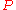, show that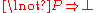or its equivalent. Then, since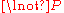implies a contradiction, conclude.

Proof by contradiction is also known as indirect proof, apagogical argument, reductio ad impossibile. It is a particular kind of the more general form of argument known as reductio ad absurdum
In logic, proof by contradiction is a form of proof that establishes the truth or validity of a proposition by showing that the proposition's being false would imply a contradiction...

.

## Examples

A classic proof by contradiction from mathematics is the proof that the square root of 2 is irrational. If it were rational, it could be expressed as a fraction a/b in lowest terms, where a and b are integers, at least one of which is odd. But if a/b = √2, then a2 = 2b2. Therefore a2 must be even.
Because the square of an odd number is odd, that in turn implies that a is even. This means that b must be odd because a/b is in lowest terms.

On the other hand, if a is even, then a2 is a multiple of 4. If a2 is a multiple of 4 and a2 = 2b2, then 2b2 is a multiple of 4, and therefore b2 is even, and so is b.

So b is odd and even, a contradiction. Therefore the initial assumption—that √2 can be expressed as a fraction—must be false.

### The length of the hypotenuse is less than the sum of the lengths of the two legs

The method of proof by contradiction has also been used to show that for any non-degenerate
Degeneracy (mathematics)
In mathematics, a degenerate case is a limiting case in which a class of object changes its nature so as to belong to another, usually simpler, class....

Right triangle
Right triangle
A right triangle or right-angled triangle is a triangle in which one angle is a right angle . The relation between the sides and angles of a right triangle is the basis for trigonometry.-Terminology:The side opposite the right angle is called the hypotenuse...

, the length of the hypotenuse is less than the sum of the lengths of the two remaining sides. The proof relies on the Pythagorean theorem
Pythagorean theorem
In mathematics, the Pythagorean theorem or Pythagoras' theorem is a relation in Euclidean geometry among the three sides of a right triangle...

. Letting c be the length of the hypotenuse and a and b the lengths of the legs, the claim is that a + b > c.
As usual, we start the proof by negating the claim and assuming that a + b ≤ c. The next step is to show that this leads to a contradiction. Squaring both sides, we have (a + b)2 ≤ c2 or, equivalently, a2 + 2ab + b2 ≤ c2. A triangle is non-degenerate if each edge has positive length, so we may assume that a and b are greater than 0. Therefore, a2 + b2 < a2 + 2ab + b2 ≤ c2. Taking out the middle term, we have a2 + b2 < c2. We know from the Pythagorean theorem that a2 + b2 = c2. We now have a contradiction since strict inequality and equality are mutually exclusive. The latter was a result of the Pythagorean theorem and the former the assumption that a + b ≤ c. The contradiction means that it is impossible for both to be true and we know that the Pythagorean theorem holds. It follows that our assumption that a + b ≤ c must be false and hence a + b > c, proving the claim.

### In mathematics

Say we wish to disprove proposition
Proposition
In logic and philosophy, the term proposition refers to either the "content" or "meaning" of a meaningful declarative sentence or the pattern of symbols, marks, or sounds that make up a meaningful declarative sentence...

p. The procedure is to show that assuming p leads to a logical contradiction
In classical logic, a contradiction consists of a logical incompatibility between two or more propositions. It occurs when the propositions, taken together, yield two conclusions which form the logical, usually opposite inversions of each other...

. Thus, according to the law of non-contradiction, p must be false.

Say instead we wish to prove proposition p. We can proceed by assuming "not p" (i.e. that p is false), and show that it leads to a logical contradiction. Thus, according to the law of non-contradiction, "not p" must be false, and so, according to the law of the excluded middle, p is true.

In symbols:

To disprove p: one uses the tautology
Tautology (logic)
In logic, a tautology is a formula which is true in every possible interpretation. Philosopher Ludwig Wittgenstein first applied the term to redundancies of propositional logic in 1921; it had been used earlier to refer to rhetorical tautologies, and continues to be used in that alternate sense...

(p → (R ∧ ¬R)) → ¬p, where R is any proposition and the ∧ symbol is taken to mean "and". Assuming p, one proves R and ¬R, and concludes from this that p → (R ∧ ¬R). This and the tautology together imply ¬p.

To prove p: one uses the tautology (¬p → (R ∧ ¬R)) → p where R is any proposition. Assuming ¬p, one proves R and ¬R, and concludes from this that ¬p → (R ∧ ¬R). This and the tautology together imply p.

For a simple example of the first kind, consider the proposition, ¬p: "there is no smallest rational number greater than 0". In a proof by contradiction, we start by assuming the opposite, p: that there is a smallest rational number, say, r0.

Now let x = r0/2. Then x is a rational number greater than 0 and less than r0. (In the above symbolic argument, "x is the smallest rational number" would be R and "r0 (which is different from x) is the smallest rational number" would be ¬R.) But that contradicts our initial assumption, p, that r0 was the smallest rational number. So we can conclude that the original proposition, ¬p, must be true — "there is no smallest rational number greater than 0".

[Note: the choice of which statement is R and which is ¬R is arbitrary.]

It is common to use this first type of argument with propositions such as the one above, concerning the non-existence of some mathematical object. One assumes that such an object exists, and then proves that this would lead to a contradiction; thus, such an object does not exist. For other examples, see proof that the square root of 2 is not rational and Cantor's diagonal argument
Cantor's diagonal argument
Cantor's diagonal argument, also called the diagonalisation argument, the diagonal slash argument or the diagonal method, was published in 1891 by Georg Cantor as a mathematical proof that there are infinite sets which cannot be put into one-to-one correspondence with the infinite set of natural...

.

On the other hand, it is also common to use arguments of the second type concerning the existence of some mathematical object. One assumes that the object doesn't exist, and then proves that this would lead to a contradiction; thus, such an object must exist. Although it is quite freely used in mathematical proofs, not every school of mathematical thought
Philosophy of mathematics
The philosophy of mathematics is the branch of philosophy that studies the philosophical assumptions, foundations, and implications of mathematics. The aim of the philosophy of mathematics is to provide an account of the nature and methodology of mathematics and to understand the place of...

accepts this kind of argument as universally valid. See further Nonconstructive proof.

### In mathematical logic

In mathematical logic
Mathematical logic
Mathematical logic is a subfield of mathematics with close connections to foundations of mathematics, theoretical computer science and philosophical logic. The field includes both the mathematical study of logic and the applications of formal logic to other areas of mathematics...

, the proof by contradiction is represented as:
If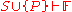thenor
If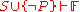then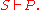In the above, P is the proposition we wish to disprove respectively prove; and S is a set of statements, which are the premise
Premise
Premise can refer to:* Premise, a claim that is a reason for, or an objection against, some other claim as part of an argument...

s — these could be, for example, the axiom
Axiom
In traditional logic, an axiom or postulate is a proposition that is not proven or demonstrated but considered either to be self-evident or to define and delimit the realm of analysis. In other words, an axiom is a logical statement that is assumed to be true...

s of the theory we are working in, or earlier theorem
Theorem
In mathematics, a theorem is a statement that has been proven on the basis of previously established statements, such as other theorems, and previously accepted statements, such as axioms...

s we can build upon. We consider P, or the negation of P, in addition to S; if this leads to a logical contradiction F, then we can conclude that the statements in S lead to the negation of P, or P itself, respectively.

Note that the set-theoretic union
Union (set theory)
In set theory, the union of a collection of sets is the set of all distinct elements in the collection. The union of a collection of sets S_1, S_2, S_3, \dots , S_n\,\! gives a set S_1 \cup S_2 \cup S_3 \cup \dots \cup S_n.- Definition :...

, in some contexts closely related to logical disjunction
Logical disjunction
In logic and mathematics, a two-place logical connective or, is a logical disjunction, also known as inclusive disjunction or alternation, that results in true whenever one or more of its operands are true. E.g. in this context, "A or B" is true if A is true, or if B is true, or if both A and B are...

(or), is used here for sets of statements in such a way that it is more related to logical conjunction
Logical conjunction
In logic and mathematics, a two-place logical operator and, also known as logical conjunction, results in true if both of its operands are true, otherwise the value of false....

(and).

## Notation

Isaac Barrow
Isaac Barrow was an English Christian theologian, and mathematician who is generally given credit for his early role in the development of infinitesimal calculus; in particular, for the discovery of the fundamental theorem of calculus. His work centered on the properties of the tangent; Barrow was...

and Baermann used the notation Q.E.A., for "quod est absurdum" ("which is absurd"), along the lines of Q.E.D.
Q.E.D.
Q.E.D. is an initialism of the Latin phrase , which translates as "which was to be demonstrated". The phrase is traditionally placed in its abbreviated form at the end of a mathematical proof or philosophical argument when what was specified in the enunciation — and in the setting-out —...

, but this notation is rarely used today. A graphical symbol sometimes used for contradictions is a downwards zigzag arrow "lightning" symbol (U+21AF: ↯), for example in Davey and Priestley. Others sometimes used include a pair of opposing arrows
Hand of Eris
The Five Fingered Hand of Eris is the name given to "two opposing arrows converging into a common point." The "canonical" example is shown at right, but the passage quoted goes on to say, "It may be vertical, horizontal, or else such, and it may be elaborated or simplified as desired."The...

(asor), struck-out arrows (), a stylized form of hash (such as U+2A33: ⨳), or the "reference mark" (U+203B: ※). The "up tack" symbol (U+22A5: ⊥) used by philosophers and logicians (see contradiction
In classical logic, a contradiction consists of a logical incompatibility between two or more propositions. It occurs when the propositions, taken together, yield two conclusions which form the logical, usually opposite inversions of each other...

) also appears, but is often avoided due to its usage for orthogonality
Orthogonality
Orthogonality occurs when two things can vary independently, they are uncorrelated, or they are perpendicular.-Mathematics:In mathematics, two vectors are orthogonal if they are perpendicular, i.e., they form a right angle...

.

## Quotations

In the words of G. H. Hardy
G. H. Hardy
Godfrey Harold “G. H.” Hardy FRS was a prominent English mathematician, known for his achievements in number theory and mathematical analysis....

(A Mathematician's Apology
A Mathematician's Apology
A Mathematician's Apology is a 1940 essay by British mathematician G. H. Hardy. It concerns the aesthetics of mathematics with some personal content, and gives the layman an insight into the mind of a working mathematician.-Summary:...

), "Reductio ad absurdum, which Euclid loved so much, is one of a mathematician's finest weapons. It is a far finer gambit than any chess
Chess
Chess is a two-player board game played on a chessboard, a square-checkered board with 64 squares arranged in an eight-by-eight grid. It is one of the world's most popular games, played by millions of people worldwide at home, in clubs, online, by correspondence, and in tournaments.Each player...

gambit
Gambit
A gambit is a chess opening in which a player, most often White, sacrifices material, usually a pawn, with the hope of achieving a resulting advantageous position. Some well-known examples are the King's Gambit , Queen's Gambit , and Evans Gambit...

: a chess player may offer the sacrifice of a pawn or even a piece, but a mathematician offers the game."